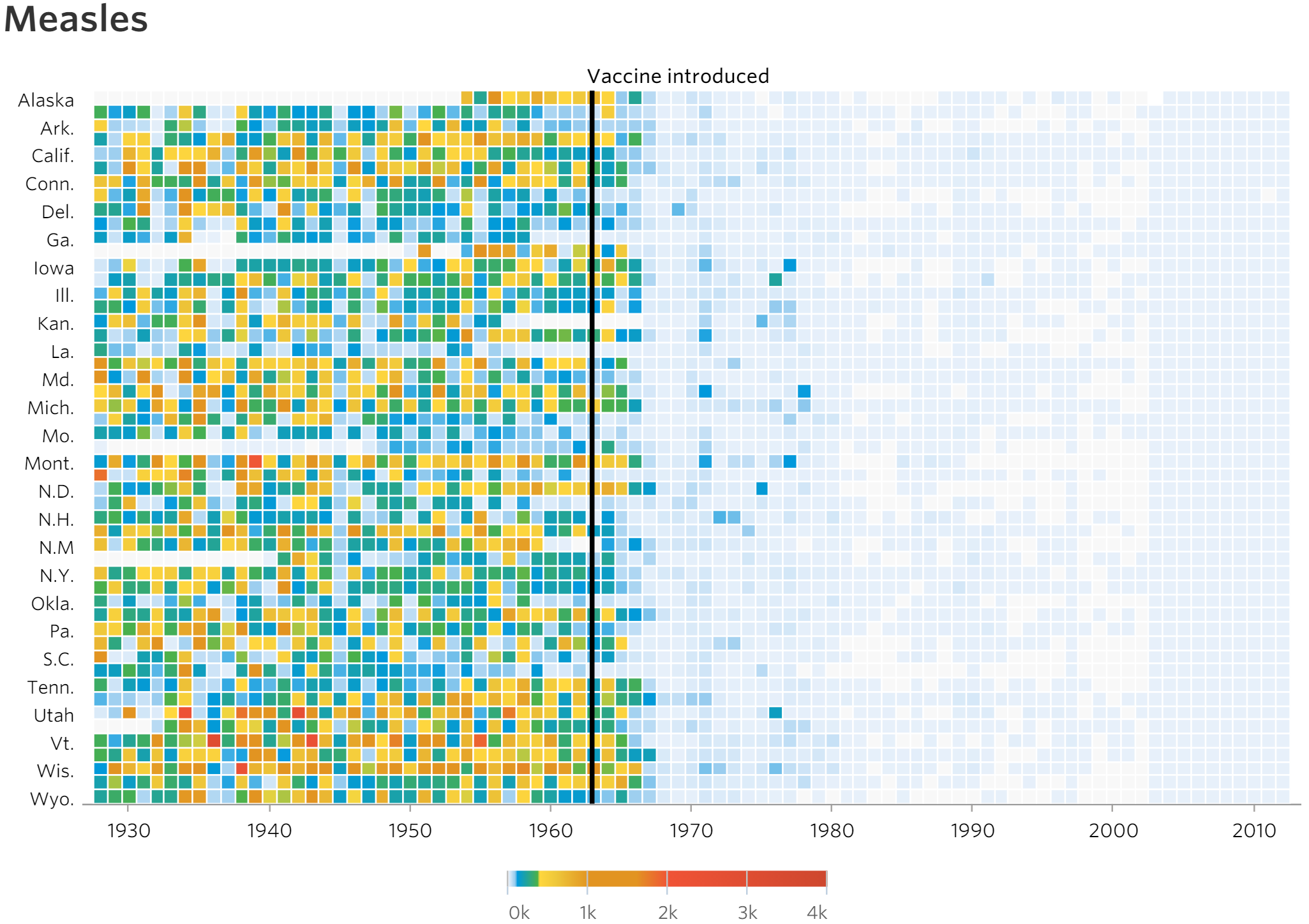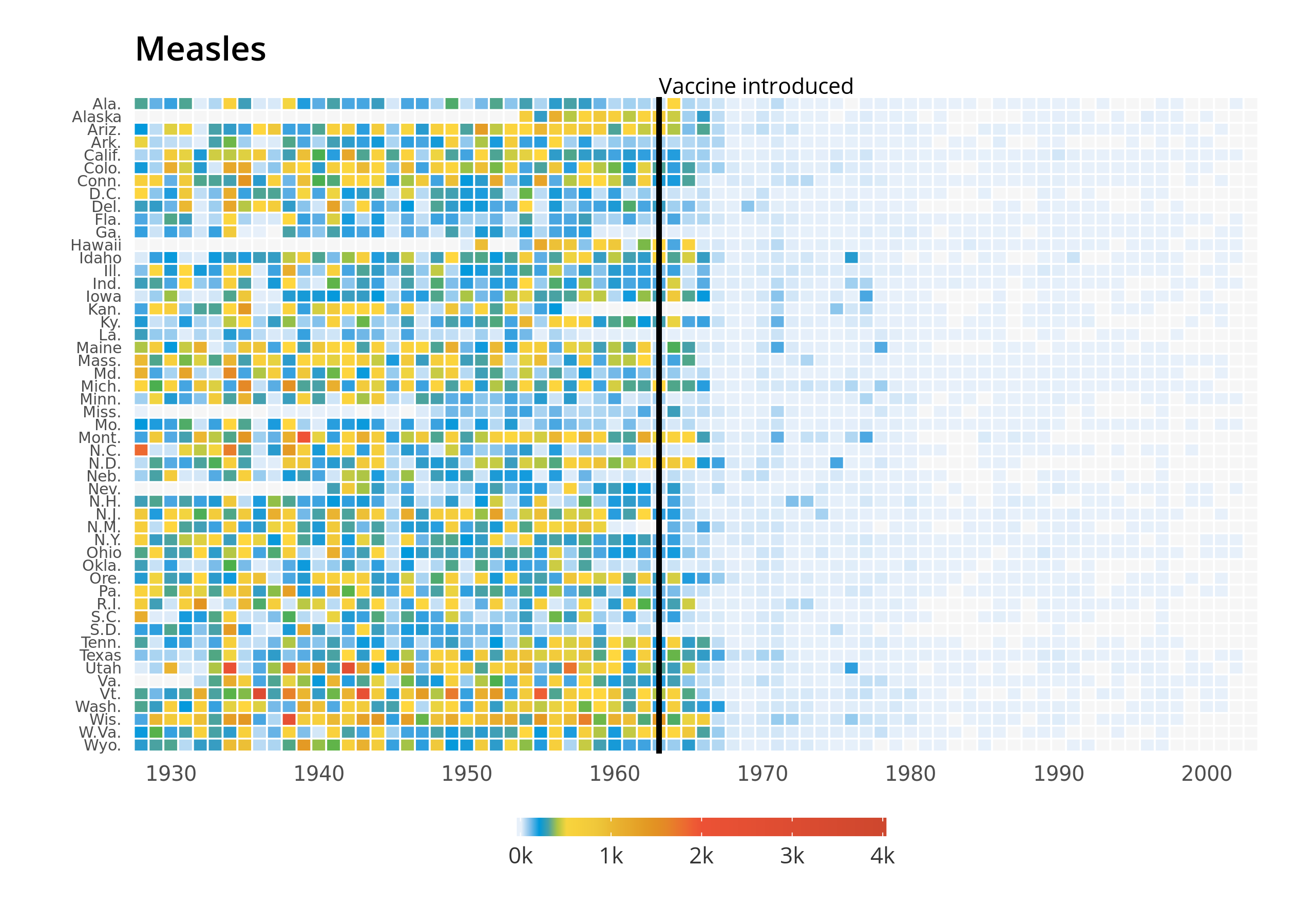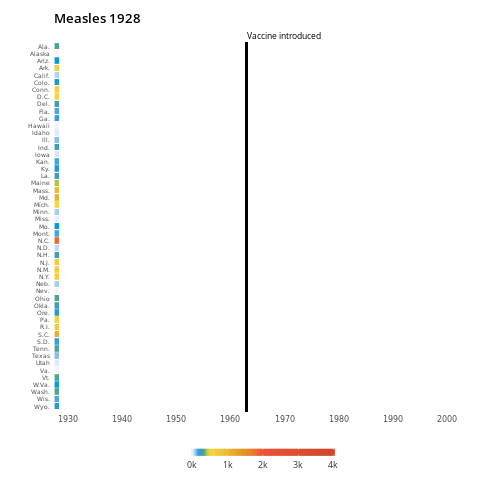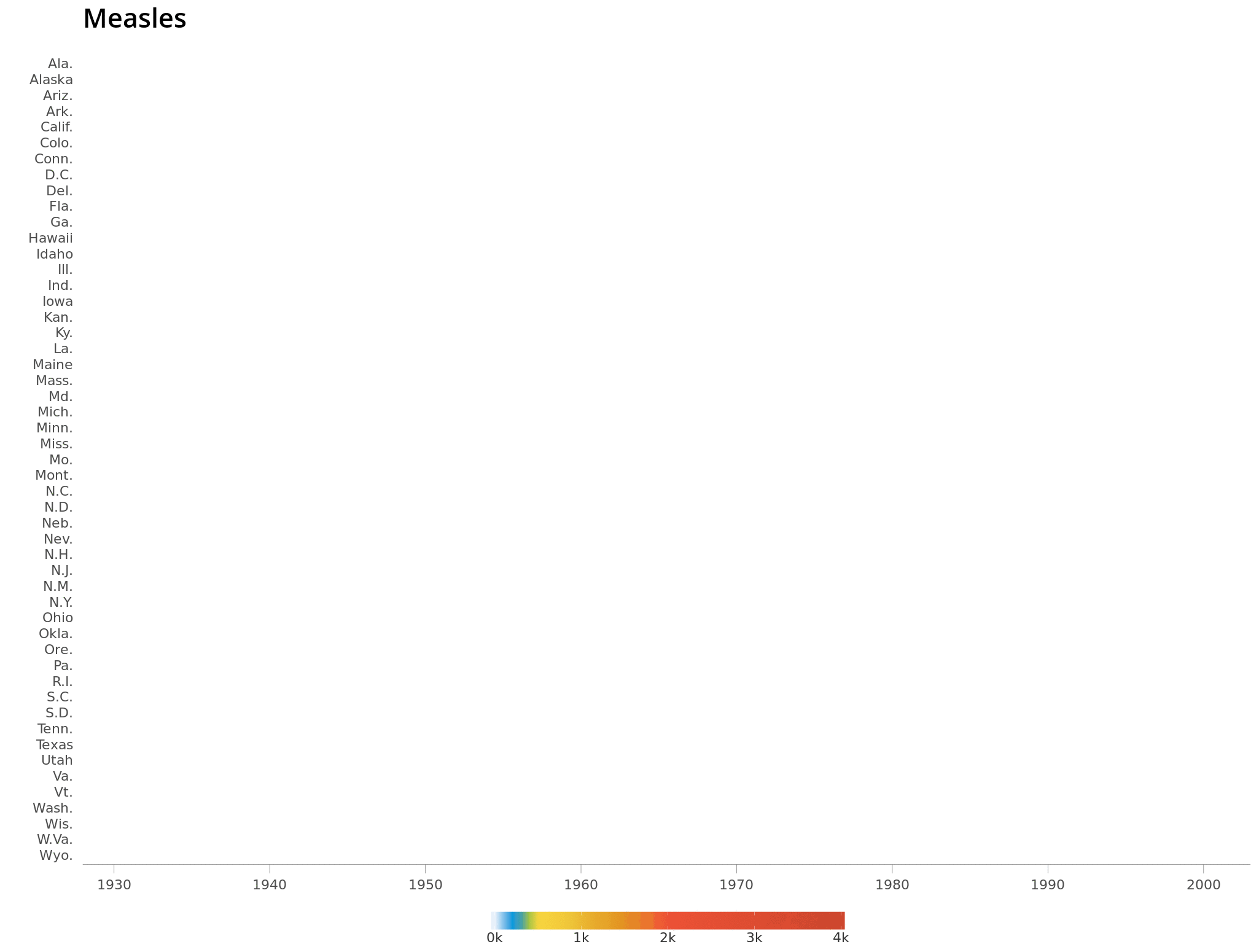# Reproducing the WSJ Measles Vaccination Chart Using R

#### June 28, 2017

Using ggplot2 and animation packages to reproduce and animate the famous Wall Street Journal Measles Incidence Chart

## Mastering Animation in R

UPDATE: In order to reproduce some portions of this code you need the `gganimate` package using the deprecated API. To access that version, download v0.1.1 here.

Recently I’ve been working on creating gif animations for social media:

It’s pretty addicting! And not as complicated as it appears. Let’s walk through the creation of a gif using R.

## Wall Street Journal Measles Vaccination Chart

One of the more compelling data visualizations produced in recent years is the following representation of measles incidence from 1928 to 2003 produced by the Wall Street Journal graphics team:Wall Street Journal

In the two years after its release, many prominent data journalists, designers, and developers have analyzed/applauded the design decisions in the chart, which urge the reader to its take-home message: the introduction of the measles vaccine drastically reduced the incidence of the virus.

Recognizing it’s merit, let’s accentuate that take-home message by adding the element of animation.

## Download dependent packages and prep the data

``````library(reshape2)
library(dplyr)
library(extrafont)
library(ggplot2)
library(gganimate)
library(animation)
library(grid)``````

We’ll use `reshape2` and `dplyr` to manipulate the data, `ggplot2` to plot it, `gganimate` for a first animation exploration, and `animation` and `grid` for a customized finished product.

## Download and prep the data (everything is a remix)

The heavy data prep and plotting for this plot has been done already! Much of this code comes from this site, with small improvements and the inclusion of some omitted pieces. From that site, I’ve relinked the steps to download the data:

1. Register and login to “Project Tycho“
2. Go to level 1 data, then Search and retrieve data
3. Now change a couple of options: geographic level := state; disease outcome := incidence
4. Add all states (highlight all at once with Ctrl+A (or Cmd+A on Macs)
5. Hit submit and scroll down to Click here to download results to excel
6. Open in excel and export to CSV

Once we’ve got the data and you’ve set your working directory, lets read in the file and reshape it:

``````measles <- read.csv("/home/michael/Documents/animation_samples/measles/measles.csv", skip=2)
measles <- melt(measles, id.var=c("YEAR", "WEEK"))
colnames(measles) <- c("year", "week", "state", "cases")
measles\$cases <- ifelse(measles\$cases=="\u002D",NA,measles\$cases)
measles\$cases <- as.numeric(measles\$cases)``````

One piece I wanted to replicate was the state abbreviations used in the figure. I used the AP style guide, and you can access that csv through this link:

``````stateAP <- read.csv("/home/michael/Documents/animation_samples/measles/stateName.csv")
stateAP\$stateName <- toupper(stateAP\$stateName)
measles\$state <- stateAP\$stateAP[match(measles\$state,stateAP\$stateName)]``````

Finally, we’ll create our measles data frame by summarizing the incidence of measles occurring in each state by year, while also recoding instances in which state’s have no incidence/values for a given year as NAs.

``````mdf <- measles %>% group_by(state, year) %>%
summarise(incidence=if(all(is.na(cases))) NA else
sum(cases, na.rm=T))
mdf\$state <- factor(mdf\$state, levels=rev(levels(mdf\$state)))``````

## Create the figure using `ggplot2`

We’ll pull the colors directly from the WSJ javascript source code. We’ll also load the Open Sans family of fonts via `loadfonts()`

``````cols<- c("#e7f0fa", #lighter than light blue
"#c9e2f6", #light blue
"#95cbee", #blue
"#0099dc", #darker blue
"#4ab04a", #green
"#ffd73e", #yellow
"#eec73a", #mustard
"#e29421", #dark khaki (?)
"#f05336", #orange red
"#ce472e") #red

Nifty!

Let’s shift to making the plot. The majority of the figure is generated via these three function calls:

``````gg <- ggplot(mdf, aes(y=state, x=year, fill=incidence)) +
geom_tile(colour="white",
width=.9, height=.9) + theme_minimal() +
values=c(0, 0.01, 0.02, 0.03, 0.09, 0.1, .15, .25, .4, .5, 1),
na.value=rgb(246, 246, 246, max=255),
labels=c("0k", "1k", "2k", "3k", "4k"),
guide=guide_colourbar(ticks=T, nbin=50,
barheight=.5, label=T,
barwidth=10))``````

The most contentious design decision made by the WSJ authors is undoubtedly the color scale. Aligning gradient color values (in our chart as `values=c(0, 0.01, 0.02, 0.03, 0.09, 0.1, .15, .25, .4, .5, 1)`) so that one portion of the scale is weighted more prominently than the other accentuates the message of the chart; some might see this as ambiguous or misleading - it’s challenging to ascribe what value a given color stands for. Once we have the skeleton for our plot, we can add an appropriate x axis scale, vaccine introduction line, and figure labels:

``````gg <- gg +
scale_x_continuous(expand=c(0,0),
breaks=seq(1930, 2010, by=10)) +
geom_segment(x=1963, xend=1963, y=0, yend=51.5, size=.9) +
labs(x="", y="", fill="") +
ggtitle("Measles")``````

Finally, we’ll add in our theme.

``````gg <- gg +
theme(legend.position=c(.5, -.13),
legend.direction="horizontal",
legend.text=element_text(colour="grey20"),
plot.margin=grid::unit(c(.5,.5,1.5,.5), "cm"),
axis.text.y=element_text(size=6, family="Open Sans Regular",
hjust=1),
axis.text.x=element_text(size=8, family="Open Sans Regular"),
axis.ticks.y=element_blank(),
panel.grid=element_blank(),
title=element_text(hjust=-.07, vjust=1,
family="Open Sans Semibold"),
text=element_text(family="Open Sans")) +
annotate("text", label="Vaccine introduced", x=1963, y=53,
vjust=1, hjust=0, size=I(3), family="Open Sans")``````

Voila!Measles Animation using animation

## Explore the `ggplot2` object with `gganimate`

It’s dang easy to create an animation using `gganimate`. Just add `frame` to the initial ggplot2 call and you’re good to go! In our plot, we’ll want to iterate over the data years, so `.frame = year`

``gganimate(gg, "/home/michael/Documents/mikeleeco.github.com/static/img/gganimate_measles.gif")``Measles Animation using gganimate

That’s a great start! Though this could be further developed using `gganimate` I prefer to use the `animation` package’s `saveGIF` function since I prefer the method in which multiple images can be called within one call.

## Refine our gif using `animation`

The main idea behind creating our animation will be iteratively printing images of larger subsets of our data set. In the for-loop, `subset(mdf, year<=1928+i-1)` takes the i-th value to subset the data year used in the figure. For example, for i==5, the data years 1928 through 1932 will appear in that image.

Besides this change, and the addition of `frame=year`, our ggplot2 call is nearly the same (I bumped up the font sizes to correspond with a larger image size). One supplement to the chart is the addition of pauses at critical points in the figure: in 1963 (when the measles vaccine was introduced) and in 2003 (the final data year of the figure). We’ll use if statements to determine whether pieces of the plot should be “drawn” given the circumstances of the subset. Here’s our final code:

``````saveGIF(
for (i in 1:76) {

gg <- ggplot(subset(mdf, year<=1928+i-1), aes(y=state, x=year, fill=incidence, frame=year)) +
geom_tile(colour="white",
width=.9, height=.9) + theme_minimal() +
values=c(0,0.01, 0.02, 0.03, 0.09, 0.1, .15, .25, .4, .5,1),
na.value=rgb(246, 246, 246, max=255),
labels=c("0k", "1k", "2k", "3k", "4k"),
guide=guide_colourbar(ticks=T, nbin=50,
barheight=2, label=T,
barwidth=40)) +
scale_x_continuous(expand=c(0,0),
breaks=seq(1930, 2010, by=10), limits = c(1928,2003))

# add in vertical line for data year >= 1963 (when the vaccine was introduced)
if(max(subset(mdf, year<=1928+i-1)\$year) >= 1963) {

gg <- gg + geom_segment(x=1963, xend=1963, y=0, yend=51.5, size=1.5) +
annotate("text", label="Vaccine introduced", x=1963, y=53,
vjust=1, hjust=0, size=I(10), family="Open Sans")
} else {
# doesn't add vertical lineif before 1963! instead annotate with text ""
gg <- gg +
annotate("text", label="", x=1963, y=53,
vjust=1, hjust=0, size=I(10), family="Open Sans")
}
gg <- gg + labs(x="", y="", fill="") +
ggtitle("Measles") +
theme(legend.position=c(.5, -.075),
legend.direction="horizontal",
legend.text=element_text(colour="grey20", size = 22, family="Open Sans Regular"),
plot.margin=grid::unit(c(.5,.5,2.5,.5), "cm"),
axis.text.y=element_text(size=22, family="Open Sans Regular",
hjust=1),
axis.text.x=element_text(size=22,family="Open Sans Regular",margin=margin(10,0,0,0,"pt")),
axis.ticks.y=element_blank(),
axis.line.x=element_line(colour = "grey50"),
axis.ticks.length=unit(.5, "cm"),
axis.ticks.x=element_line(colour = "grey50"),
panel.grid=element_blank(),
title=element_text(hjust=-.07, vjust=1, size = 36,
family="Open Sans Semibold"),
text=element_text(family="Open Sans"))

# adding in pauses for data year == 1963 (when the vaccine was introduced) and 2003 (the last year in our data)
# the replicate() function will take a ggplot2 object and print it n-times via grid.draw()
if(max(subset(mdf, year<=1928+i-1)\$year) == 1963) {
replicate(30,grid.draw(gg))
} else if (max(subset(mdf, year<=1928+i-1)\$year) == 2003) {
replicate(30,grid.draw(gg))
} else {
print(gg)
}
}
,movie.name="/home/michael/Documents/mikeleeco.github.com/static/img/measles.gif",interval = .1, ani.width = 2050, ani.height = 1550)``````Measles Animation using animation

### Addendum - Gif File Size Management:

Customized gifs can get super large. The version above tops of at 73.4 MB! We’ll need to reduce that size for . One source for an #rstats solution is this site - which uses the open source tool gifsicle to reduce gif file sizes. Let’s port this author’s `gif_compress` script to minimize our measles gif:

``````gif_compress <- function(ingif, outgif, show=TRUE, extra.opts=""){
command <-  sprintf("gifsicle -O3 %s < %s > %s", extra.opts, ingif, outgif)
system.fun <- if (.Platform\$OS.type == "windows") shell else system
if(show) message("Executing: ", strwrap(command, exdent = 2, prefix = "\n"))
system.fun(ifelse(.Platform\$OS.type == "windows", sprintf("\"%s\"", shQuote(command)), command))
}
gif_compress("/home/michael/Documents/mikeleeco.github.com/static/img/measles.gif","/home/michael/Documents/mikeleeco.github.com/static/img/measlesCompressed.gif",extra.opts="--colors 256")

file.size("/home/michael/Documents/mikeleeco.github.com/static/img/measles.gif")``````
``##  NA``
``file.size("/home/michael/Documents/mikeleeco.github.com/static/img/measlesCompressed.gif")``
``##  NA``

Still large but more manageable.

Interested in learning more? Hire me to consult on your next project, follow me on twitter, leave a comment, or contact me via email. All inquiries welcome!